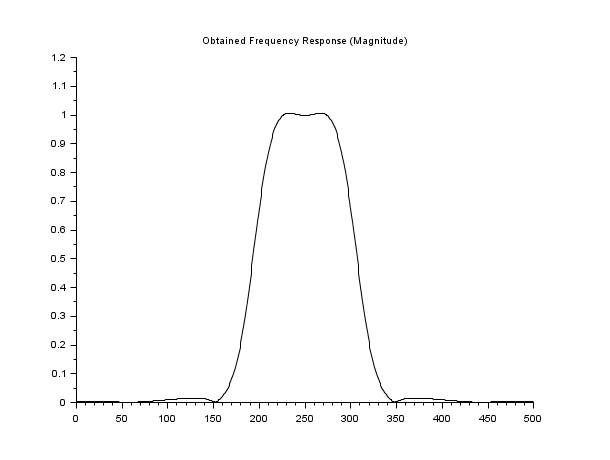Scilab Home page | Wiki | Bug tracker | Forge | Mailing list archives | ATOMS | File exchange
Change language to: Français - Português - 日本語 - Русский

See the recommended documentation of this function

Scilab Help >> Signal Processing > Filters > yulewalk

# yulewalk

least-square filter design

### Syntax

`Hz = yulewalk(N,frq,mag)`

### Arguments

N

integer (order of desired filter)

frq

real row vector (non-decreasing order), frequencies.

mag

non negative real row vector (same size as frq), desired magnitudes.

Hz

filter `B(z)/A(z)`

### Description

Hz = yulewalk(N,frq,mag) finds the N-th order iir filter

```n-1         n-2
B(z)   b(1)z     + b(2)z    + .... + b(n)
H(z)= ---- = ---------------------------------
n-1       n-2
A(z)    z   + a(2)z    + .... + a(n)```

which matches the magnitude frequency response given by vectors frq and mag. Vectors frq and mag specify the frequency and magnitude of the desired frequency response. The frequencies in frq must be between 0.0 and 1.0, with 1.0 corresponding to half the sample rate. They must be in increasing order and start with 0.0 and end with 1.0.

### Examples

```f=[0,0.4,0.4,0.6,0.6,1];
H=[0,0,1,1,0,0];
Hz=yulewalk(8,f,H);
fs=1000;
fhz = f*fs/2;
scf(0);
clf
plot2d(fhz',H');
xtitle('Desired Frequency Response (Magnitude)')
[frq,repf]=repfreq(Hz,0:0.001:0.5);
scf(1);
clf
plot2d(fs*frq',abs(repf'));
xtitle('Obtained Frequency Response (Magnitude)')```